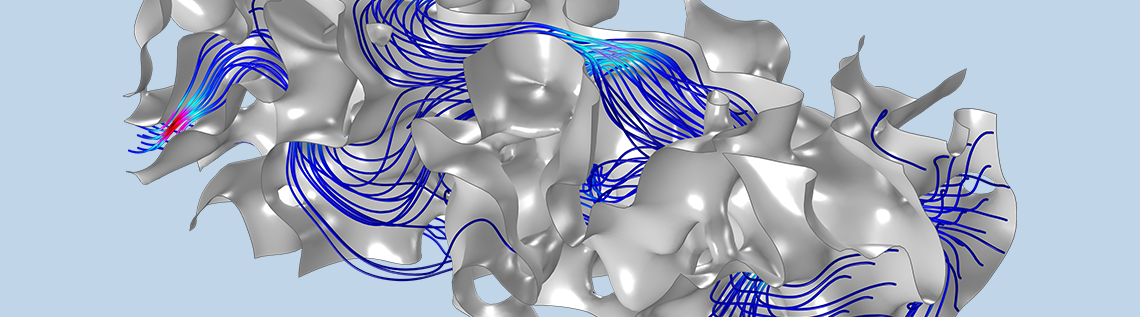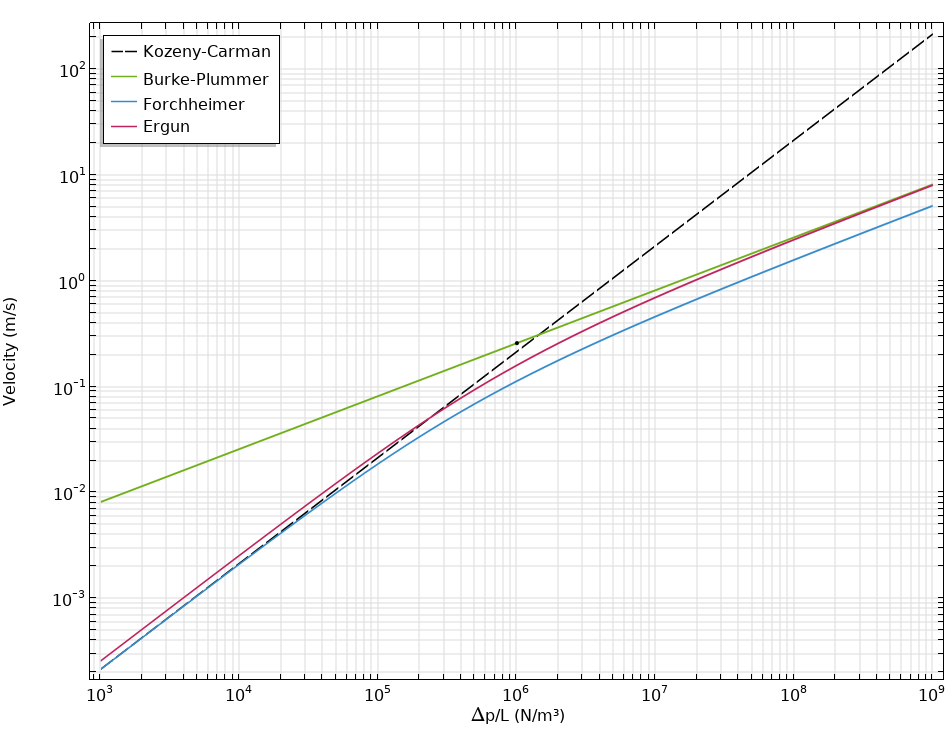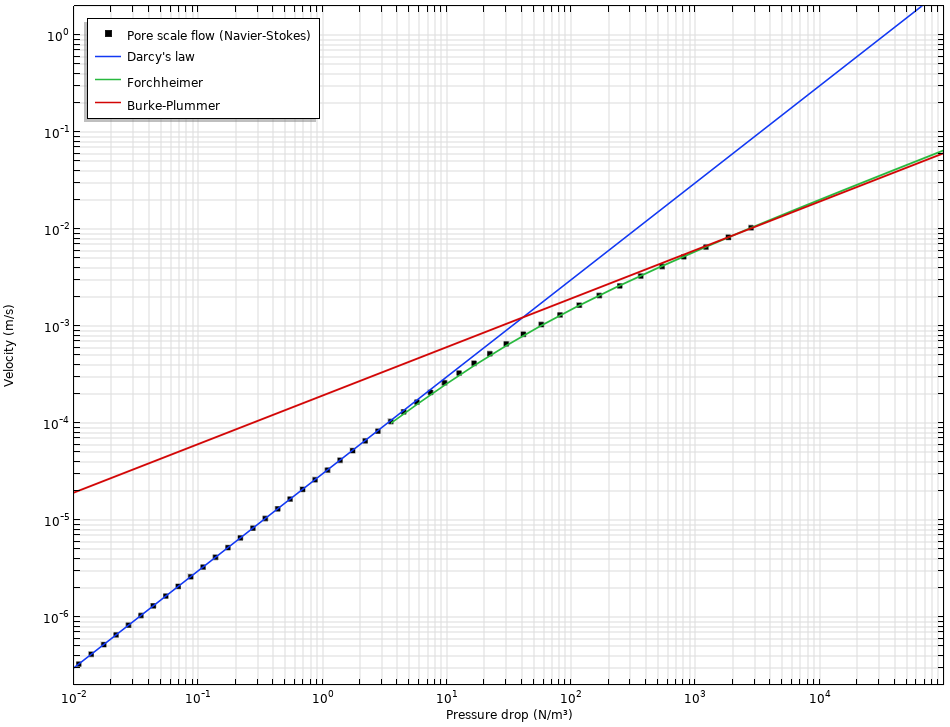# 模拟多孔介质中的达西流和非达西流

2020年 2月 24日

### 在微观尺度上模拟多孔介质中的流动

• 孔隙率 \epsilon_p=\frac{V_\textrm{pore}}{V_\textrm{tot}}，描述了孔隙体积与总体积的比率，可以从几何形状计算
• 沿流动方向（纵向）下降的压力 \Delta p/L，可以计算或预定义
• 表观速度 u=\frac{Q} {A}，或通过结构的体积流量 Q (m3/s)，除以总横截面积 A(m2 )

### 宏观尺度的流动

(1)

\mathbf{u}=-\frac{\kappa}{\mu}\nabla p

(2)

\kappa=\frac{d_\textrm{p}^2}{180}\frac{\epsilon_p^3}{(1-\epsilon_p)^2}

(3)

Re=\frac{\rho u L}{\mu}

-\nabla p = \frac{\mu}{\kappa}\mathbf{u}+\beta\rho\mathbf{u}|\mathbf{u}|

(4)

\beta=\frac{c_F}{\sqrt{\kappa}}

(5)Kozeny-CarmanForchheimerErgun Burke-Plummer 关系的比较。

(6)

\kappa=\kappa_\infty\left(1+\frac{b_\textrm{K}}{p_\textrm{A}}\right)

Klinkenberg 参数 b_\textrm{K} (Pa) 取决于多孔介质的渗透率，我们可以在文献中查到 b_\textrm{K}
\propto\kappa_\infty^{-0.36}COMSOL 中的多孔介质流模块包含了所有上述渗透率模型。Forchheimer 和 Kozeny-Carman 方程也可用于支持多孔介质流动的其他模块。

### 非达西流，从微观到宏观尺度Forchheimer 方程可以很好地计算过渡区域。在本文的示例中，将 Forchheimer 方程与来自微观模型的数据相拟合，以获得 Forchheimer 参数 c_\textrm{F}，该数据通常是在实验中确定的。

### 参考文献

1. Y. Wu, K. Pruess, and P. Persoff, “Gas Flow in Porous Media With Klinkenberg Effects“, Transport in Porous Media, vol. 32, pp. 117–137, 1998.
2. J. Bear, Dynamics of Fluids in Porous Media, Courier Corporation, 1988.